Equations for Photovoltaics

Basic Equations

Density of States in Conduction and Valence Band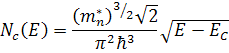Fermi function: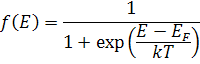Carrier Concentration in Equilibrium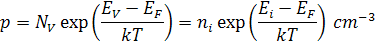Law of mass action:Carrier concentrations:

n-type material: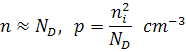p-type material: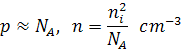Carrier Concentration Under Bias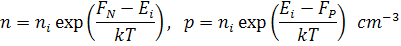Generation

Number of photons: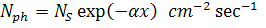Generation rate:Generation, homogeneous semiconductor: G = const:

P-type: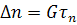N-type: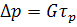Recombination

General SRH recombination rate: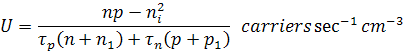Under low injection conditions:

For electrons:For holes: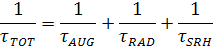Basic PN Junction Equation Set

1. Poisson's equaion: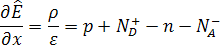2. Transport equations: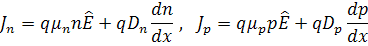3. Continuity equations: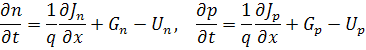General solution for no electric eifled, constant generation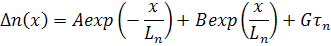Equations for PN Junctions

Built-in voltage pn homojunction:General ideal diode equation: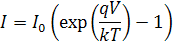I0 for wide base diode: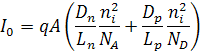I0 for narrow base diode: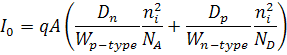Full diode saturation currrent equation: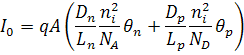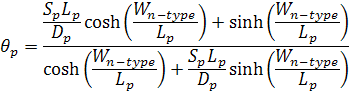Depletion region recombination: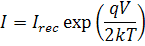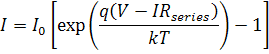Solar Cell Equations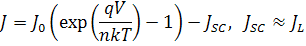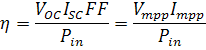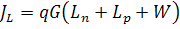for constant G, wide base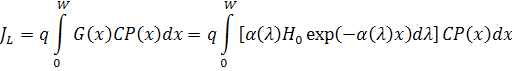Material Constants and Commont Units

Intrinsic carrier concentration: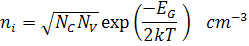Effective density of states: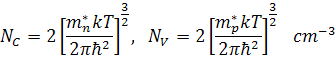Intrinsic energy level:Diffusivity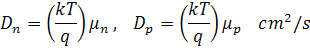Minority carrier diffusion length: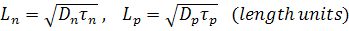Resistivity and conductivity: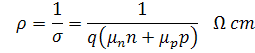Resistance, homogeneous: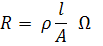Permittivity:Wavelength and energy of a photon: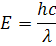If E is in eV and λ is in μm:Spectral irradiance for black body: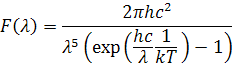Power density of a non-ideal black body:Photon flux and power density:Material mn*/m0 mp*/m0 EG (eV) ni (cm-3) c (eV) eS Si 1.18 0.81 1.12 1.0 × 1010 4.03 11.8 Ge 0.55 0.36 0.66 2.0 × 1013 4.13 16.0 GaAs 0.066 0.52 1.42 1.8 × 106 4.07 13.1 InP 0.08 0.6 1.34 1.3 × 107 4.38 12.5